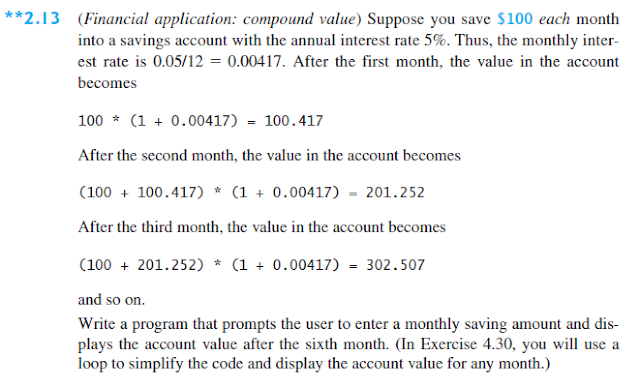# Financial Application: Compound Value

The program below is the answer to Liang's Introduction to Java Programming (9th Edition) Chapter 2 Exercise 2.13.```/**
*
* @Author: Aghatise Osazuwa
* Website: www.cscprogrammingtutorials.com
*
* Exercise 2.13 - Financial Application: Compound Value
*
*/

import java.util.Scanner;

public class CompoundValue {

public static void main(String[] args) {

//Display Program Information
System.out.println("This Program Calculates The Compound Value Of An "
+ "Account After The Sixth Month.\n");

//create Scanner
Scanner input = new Scanner(System.in);

//prompt user to enter details
System.out.println("Enter the monthly saving amount:");
double savings = input.nextDouble();

//calculate the account value after the sixth month
double firstMonth = savings * (1 + 0.00417);
double secondMonth = (savings + firstMonth) * (1 + 0.00417);
double thirdMonth = (savings + secondMonth) * (1 + 0.00417);
double fourthMonth = (savings + thirdMonth) * (1 + 0.00417);
double fifthMonth = (savings + fourthMonth) * (1 + 0.00417);
double sixthMonth = (savings + fifthMonth) * (1 + 0.00417);

//format the sixth month to two decimal places
sixthMonth = (int) (sixthMonth * 100) / 100.0;

//display the result
System.out.println("After the sixth month, the account value is \$"
+ sixthMonth + "\n");
}
}
```

Click here to see other solutions to Introduction to Java Programming.